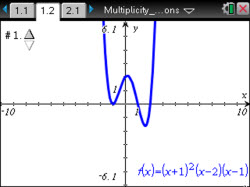# Activities

••• ##### Subject Area

• Math: Precalculus: Polynomial, Power, and Rational Functions

• ##### Author9-12

45 Minutes

• ##### Device
•TI-Nspire™ CX/CX II
•TI-Nspire™ CX CAS/CX II CAS
• TI-Nspire™ Navigator™
• TI-Nspire™
• TI-Nspire™ CAS
• ##### Software

TI-Nspire™
TI-Nspire™ CAS

3.0

## Multiplicity of Zeros of Functions#### Activity Overview

Students will utilize graphs and equations of five polynomial functions to determine the zeros of the functions and whether the functions cross the x-axis at these zeros or just touch the x-axis at the zeros. Then students will determine the degree of the polynomial functions and the effect the degree has upon the end behavior of the functions.

#### Objectives

• Students will determine the multiplicity of zeros of a polynomial function when given its graph or its equation in factored form.
• Students will write an equation for a polynomial function when given information about its zeros and the multiplicity of the zeros.
• Students will write an equation for a polynomial function when given its graph.
• Use appropriate tools strategically. (CCSS Mathematical Practice)
• Look for and make use of structure. (CCSS Mathematical Practice)

#### Vocabulary

This lesson involves students utilizing graphs and equations of five polynomial functions to determine the zeros of the functions and whether the functions cross the x-axis at these zeros or just touch the x-axis at the zeros.

As a result, students will:

• determine the degree of the polynomial functions and the effect the degree has upon the end behavior of the functions.
• write possible equations for a polynomial function when given information about its zeros.
• write the equations in factored form, given the graphs of three functions.